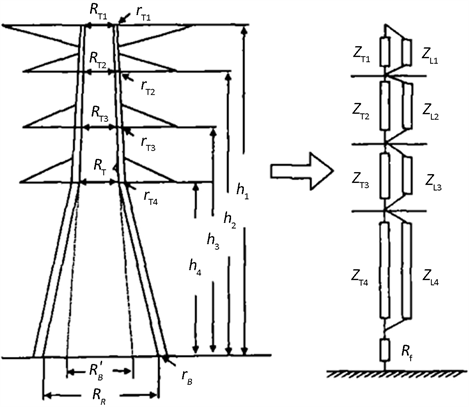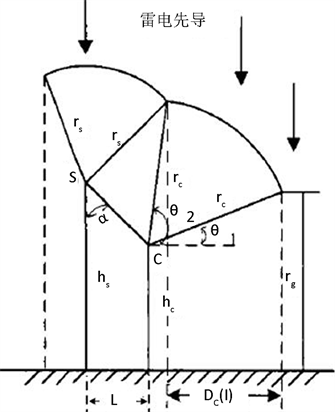# 山东地区差异化防雷研究Study on Differentiation Lightning Protection in Shandong

• 全文下载: PDF(1034KB)    PP.586-594   DOI: 10.12677/SG.2018.86065
• 下载量: 159  浏览量: 613   科研立项经费支持

Based on the influence of factors such as complicated geomorphology and obvious climatic differ-ences on lightning protection design in Shandong, this paper studies the differentiation lightning protection design of overhead-line in Shandong based on the improved electro-geometric model proposed by Eriksson, and summarizes the mathematical model suitable for Lightning Trip Rate calculation in Shandong.

1. 引言

2. 地区不同雷电活动影响

IEEE 1243-1997推荐地闪密度可以利用公式(1)来计算。

${N}_{g}=0.04{T}_{d}^{1.25}$ (1)

$\left\{\begin{array}{l}N={N}_{g}\frac{b+4{h}_{g}}{1000}×100,\left({U}_{n}<1000\text{\hspace{0.17em}}\text{kV}\right)\\ N={N}_{g}\frac{b+28{h}_{g}^{0.6}}{1000}×100,\left({U}_{n}>1000\text{\hspace{0.17em}}\text{kV}\right)\end{array}$ (2)

$\left\{\begin{array}{l}\mathrm{lg}P=-\frac{I}{C}\\ f\left(I\right)=\frac{\mathrm{ln}10}{C}{10}^{-\frac{I}{C}}\end{array}$ (3)

IEEE推荐的雷电流幅值分布函数的计算方法以及相应的雷电流幅值密度函数的计算方法如式(4)所示。

$\left\{\begin{array}{l}P=\frac{1}{1+{\left(\frac{I}{31}\right)}^{2.6}}\\ f\left(I\right)=\frac{2.6{I}^{1.6}}{{31}^{2.6}{\left[1+{\left(\frac{I}{31}\right)}^{2.6}\right]}^{2}}\end{array}$ (4)Table 1. The discipline method is compared with the IEEE recommended formula

3. 反击跳闸率影响因素及其计算

3.1. 反击跳闸率的计算

${n}_{1}=N\left(1-{P}_{a}\right)g{P}_{1}\eta$ (5)Table 2. Different terrain Striking Rate

3.2. 线路耐雷水平的计算

${I}_{1}=\frac{{u}_{50%}}{\left(1-k\right)\beta {R}_{i}+\left(\frac{{h}_{a}}{{h}_{t}}-k\right)\beta \frac{{L}_{t}}{{T}_{1}}+\left(1-\frac{{h}_{g}}{{h}_{c}}{k}_{0}\right)\frac{{h}_{c}}{{T}_{1}}}$ (6)Table 3. Tower shunt coefficient

$\eta =\left(4.5{E}^{0.75}-14\right)×{10}^{-2}$ (7)

$E=\frac{{U}_{n}}{\sqrt{3}{l}_{i}}$ (8)

$E=\frac{{U}_{n}}{2{l}_{i}+{l}_{m}}$ (9)Table 4. Corona correction factor for lightning strikes the top of the tower

3.3. 电磁环境对防雷设计的影响

${Z}_{ii}=\frac{{\alpha }_{ii}}{\nu }=60\mathrm{ln}\frac{2{h}_{i}}{{r}_{eq}}$ (10)

${Z}_{ij}=\frac{{\alpha }_{ij}}{\nu }=60\mathrm{ln}\frac{2{H}_{ij}}{{D}_{ij}}$ (11)

${Z}_{c}=\frac{{Z}_{0}}{\frac{{E}_{0}}{u}\left(1-\frac{6\sqrt{M}}{7}\right)+\frac{6\sqrt{M}}{7}\sqrt{\frac{{E}_{0}}{u}}}$ (12)

${E}_{d0}=nr{E}_{c}\mathrm{ln}\left(\frac{2h}{{r}_{eq}}\right){\left[1+\frac{r\left(n-1\right)}{A}\right]}^{-1}$ (13)

${E}_{c}=30m\delta \left(1+\frac{0.308}{\sqrt{\delta r}}\right)$ (14)

$A=\frac{d}{2\mathrm{sin}\frac{n}{\text{π}}}$ (15)

${r}_{eq}=\sqrt[n]{nr{A}^{n-1}}$ (16)

${E}_{b0}=\frac{3000m\left(1+\frac{0.03}{\sqrt{{r}_{b}}}\right)2{r}_{b}}{1+\frac{2{r}_{b}}{D}}\mathrm{ln}\left(\frac{2{h}_{b}}{\sqrt{{r}_{b}D}}\right)$ (17)

${k}_{m-1,m}=-\frac{{Y}_{m1}+{Y}_{m2}+\cdots +{Y}_{mm}}{{Y}_{mm}}$ (18)

$Z=60\left(\mathrm{ln}\frac{\sqrt{2}ct}{r}-2\right)$ (19)

$Z=60\left(\mathrm{ln}\frac{2\sqrt{2}h}{r}-2\right)$ (20)Figure 1. Multi-wave impedance model diagram

${Z}_{tk}=60\left(\mathrm{ln}\frac{{2}^{\frac{2}{3}}{h}_{k}}{{r}_{ek}}-2\right)$ (21)

${r}_{ek}={2}^{\frac{1}{8}}{\left({r}_{tk}^{\frac{1}{3}}{r}_{B}^{\frac{2}{3}}\right)}^{\frac{1}{4}}{\left({R}_{tk}^{\frac{1}{3}}{R}_{B}^{\frac{2}{3}}\right)}^{\frac{3}{4}}$ (22)

${Z}_{Lk}=9{Z}_{tk}$ (23)

4. 绕击率影响因素及其差异化计算

Eriksson提出的计算方法如公式(24)所示。

${R}_{c}=0.67{h}^{0.6}{I}^{0.74}$ (24)Figure 2. Schematic diagram of conventional lightning strike model

${R}_{e}=\left(0.36+0.168\mathrm{ln}\left(43-h\right)\right){R}_{c}$ (25)

${R}_{e}=0.55{R}_{c}$ (26)

${D}_{c}\left(I\right)={r}_{c}\left(\mathrm{cos}{\theta }_{1}-\mathrm{cos}{\theta }_{2}\right)$ (27)

$\left\{\begin{array}{l}\text{SFFOR}=2{N}_{g}L{\int }_{I={I}_{c}}^{I={I}_{\mathrm{max}}}{D}_{C}\left(I\right)f\left(I\right)\text{d}I\\ \text{SFTR}=\text{SFFOR}×\eta \end{array}$ (28)

${I}_{c}=\frac{2×{U}_{50%}}{{Z}_{c}}$ (29)

$\stackrel{¯}{SC}=\sqrt{{L}^{2}+{\left({h}_{s}-{h}_{c}\right)}^{2}}$ (30)

${\theta }_{1}=\mathrm{arcsin}\left(\frac{{r}_{g}-{h}_{c}}{{r}_{c}}\right)$ (31)

${\theta }_{2}=\frac{\text{π}}{2}+\alpha -\mathrm{arccos}\left(\frac{{r}_{c}^{2}+{\left(\stackrel{¯}{SC}\right)}^{2}-{r}_{s}^{2}}{2{r}_{c}\stackrel{¯}{SC}}\right)$ (32)

$\alpha =\mathrm{arctan}\frac{L}{{h}_{s}-{h}_{c}}$ (33)

5. 结语

  陈家宏, 赵淳, 谷山强, 等. 我国电网雷电监测与防护技术现状及发展趋势[J]. 高电压技术, 2016, 42(11): 3361-3375.  Brown, G.W. and Whitehead, E.R. (1969) Field and Analytical Studies of Transmission Line Shielding: Part II. IEEE Transactions on Power Apparatus and Systems, 88, 617-625. https://doi.org/10.1109/TPAS.1969.292350  许飞, 王建国, 周文俊, 等. 改进电气几何模型对输电线路绕击率的计算及典型故障分析[J]. 高压电器, 2006, 42(3): 205-207.  唐巧玲, 孙荆茶, 孙迎春. 山东省闪电分布及雷电灾害特征分析[J]. 现代电子技术, 2012, 35(2): 65-68.  马红松, 张立波. 山东省雷电灾害分析及雷电防御[J]. 山东气象, 2005, 25(103): 46-47.  左迎芝, 朱睦正, 张文煌. 山东地区不同区域闪电与湿度的相关分析[J]. 兰州大学学报(自然科学版), 2009(45): 72-75.  于怀征, 张民凯, 陈志超, 等. 山东省闪电活动与降水关系的研究[J]. 现代农业科技, 2009(20): 355, 357.  唐巧玲. 山东省雷电活动特征及雷电灾害风险区划研究[D]: [硕士学位论文]. 兰州: 兰州大学, 2013.  Hara, T. and Yamamoto, Q. (1996) Modeling of a Transmission Tower for Lightning Surge Analysis. IEE Proceedings—Generation, Transmission and Distribution, 143, 283-289.  廖民传, 汪晶毅, 李志泰, 等. 混压同塔多回输电线路绕击建模与评估[J]. 电网技术, 2013, 37(9): 2547-2552.